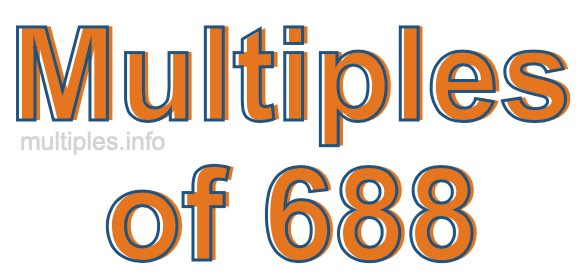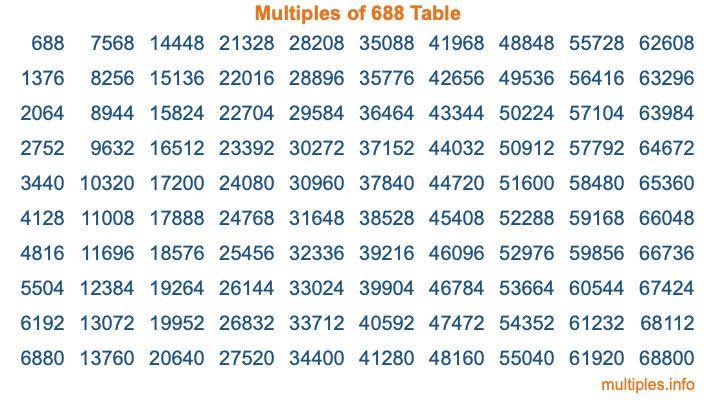Multiples of 688Welcome to the Multiples of 688 page. Here we will first teach you everything you will ever need to know about the multiples of 688, and then give you a study guide summary of everything we taught you to make sure you remember it all. Use this page to look up facts and learn information about the multiples of 688. This page will make you a multiples of six hundred eighty-eight expert!

Definition of Multiples of 688
Multiples of 688 are all the numbers that when divided by 688 equal an integer. Each of the multiples of 688 are called a multiple. A multiple of 688 is created by multiplying 688 by an integer.

Therefore, to create a list of multiples of 688, you start with 1 multiplied by 688, then 2 multiplied by 688, then 3 multiplied by 688, and so on for as long as you want. Thus, the list of the first five multiples of 688 is 688, 1376, 2064, 2752, and 3440. To see a larger list of multiples of 688, see the printable image of Multiples of 688 further down on this page. We also have a category where you can choose any nth multiple of 688.

Multiples of 688 Checker
The Multiples of 688 Checker below checks to see if any number of your choice is a multiple of 688. In other words, it checks to see if there is any number (integer) that when multiplied by 688 will equal your number. To do that, we divide your number by 688. If the the quotient is an integer, then your number is a multiple of 688.

Is  a multiple of 688?

Least Common Multiple of 688 and ...
A Least Common Multiple (LCM) is the lowest multiple that two or more numbers have in common. This is also called the smallest common multiple or lowest common multiple and is useful to know when you are adding our subtracting fractions. Enter one or more numbers below (688 is already entered) to find the LCM.

Check out our LCM Calculator if you need more details about the Least Common Multiple or if you need the LCM for different numbers for adding and subtraction fractions.

nth Multiple of 688
As we stated above, 688 is the first multiple of 688, 1376 is the second multiple of 688, 2064 is the third multiple of 688, and so on. Enter a number below to find the nth multiple of 688.

th multiple of 688

Multiples of 688 vs Factors of 688
688 is a multiple of 688 and a factor of 688, but that is where the similarities end. All postive multiples of 688 are 688 or greater than 688. All positive factors of 688 are 688 or less than 688.

Below is the beginning list of multiples of 688 and the factors of 688 so you can compare:

Multiples of 688: 688, 1376, 2064, 2752, 3440, etc.

Factors of 688: 1, 2, 4, 8, 16, 43, 86, 172, 344, 688

As you can see, the multiples of 688 are all the numbers that you can divide by 688 to get a whole number. The factors of 688, on the other hand, are all the whole numbers that you can multiply by another whole number to get 688.

It's also interesting to note that if a number (x) is a factor of 688, then 688 will also be a multiple of that number (x).

Multiples of 688 vs Divisors of 688
The divisors of 688 are all the integers that 688 can be divided by evenly. Below is a list of the divisors of 688.

Divisors of 688: 1, 2, 4, 8, 16, 43, 86, 172, 344, 688

The interesting thing to note here is that if you take any multiple of 688 and divide it by a divisor of 688, you will see that the quotient is an integer.

Multiples of 688 Table
Below is an image of the first 100 multiples of 688 in a table. The table is in chronological order, column by column. The first column has the first ten multiples of 688, the second column has the next ten multiples of 688, and so on.The Multiples of 688 Table is also referred to as the 688 Times Table or Times Table of 688. You are welcome to print out our table for your studies.

Negative Multiples of 688
Although not often discussed or needed in math, it is worth mentioning that you can make a list of negative multiples of 688 by multiplying 688 by -1, then by -2, then by -3, and so on, to get the following list of negative multiples of 688:

-688, -1376, -2064, -2752, -3440, etc.

Multiples of 688 Summary
Below is a summary of important Multiples of 688 facts that we have discussed on this page. To retain the knowledge on this page, we recommend that you read through the summary and explain to yourself or a study partner why they hold true.

There are an infinite number of multiples of 688.

A multiple of 688 divided by 688 will equal a whole number.

688 divided by a factor of 688 equals a divisor of 688.

The nth multiple of 688 is n times 688.

The largest factor of 688 is equal to the first positive multiple of 688.

688 is a multiple of every factor of 688.

688 is a multiple of 688.

A multiple of 688 divided by a divisor of 688 equals an integer.

688 divided by a divisor of 688 equals a factor of 688.

Any integer times 688 will equal a multiple of 688.

Multiples of a Number
Here you can get the multiples of another number, all with the same attention to detail as we did for multiples of 688 on this page.

Multiples of
Multiples of 689
Did you find our page about multiples of six hundred eighty-eight educational? Do you want more knowledge? Check out the multiples of the next number on our list!